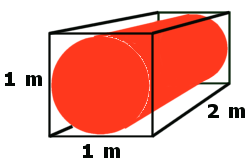## Areas & VolumesNew Topic: Surface Area & Volume, MiA 4³ Ch1 In this topic you will learn how to calculate the surface area and volume of prisms and composite solids.

### 7 Responses

1. Thursday 26 August, period 1

Revision of Area calculations.
First consider basic shapes such as rectangle, triangle and circle.
Use triangles to calculate areas of quadrilaterals.
Emphasise setting down, labelling and appropriate rounding/units.

2. Friday 27 August, period 6

Areas of composite shapes.
Worked examples followed by Ex6.1

3. Tuesday 31 August, period 3

Volumes of Prisms
Definition of a prism then derive method for finding volume.

Worked examples for cuboid, triangular prism, cylinder and generic prism.
Ex7.1

4. Wednesday 1 September, period 1

Surface Areas
Worked examples then problems from Ex10.1

5. Thursday 2 September, period 1

Complete Ex10.1 – Surface Area & Volume problems then look at exam questions

6. Friday 3 September, period 6

Go over Proportion & Variation homework.
Revise non-calculator exam questions (page 1)

7. Homework discussed and model answers copied into jotters. Some pupils will resubmit similar questions instead of corrections.

Worked on more A&V exam questions instead of non-calc.

Set p268 Q4(a) and Q5 as homework for Wednesday.